# Nonparametric and Cox-based estimation of average treatment effects in competing risks using ‘causalCmprsk’ package

#### 2023-03-28

The causalCmprsk package is designed for estimation of average treatment effects (ATE) of point interventions on time-to-event outcomes with $$K\geq 1$$ competing events. We will illustrate how to use causalCmprsk package by analyzing data from an observational study on right heart catheterization (RHC) procedure, collected by Connors et al. (1996).

# Why causalCmprsk?

• It implements both nonparametric estimation (based on the weighted Aalen-Johansen estimator) which does not assume any model for potential outcomes, and Cox-based estimation that relies on the proportional hazards assumption for potential outcomes.

• It provides three measures of treatment effect on time-to-event outcomes: cause-specific hazard ratios which are time-dependent measures under a nonparametric model, risk-based measures such as cause-specific risk differences and cause-specific risk ratios, and restricted-mean-time differences which quantify how much time on average was lost (or gained) due to treatment by some specified time point. Restricted-mean-time difference is an intuitive summary of treatment effects that translates the treatment effect from the probability (risk) into the time domain and allows to quantify, for example, by how many days or months the treatment speeds up the recovery or postpones death.

• It implements different weights representing different target populations. ATE and stab.ATE weights aim to estimate average treatment effects for the original population. ATT weights target average treatment effects for the population of treated, and ATC weights correspond to the population of untreated. overlap weights aim to estimate average treatment effects for the overlap population, that is the population with the largest equipoise regarding the treatment.

• It uses Bayesian bootstrap for estimation of uncertainty. Bayesian bootstrap is considered a more stable alternative to the simple bootstrap for time-to-event data. We implemented parallel computing to speed up bootstrap replications.

• It can be used for a Randomized Controlled Trials setting when there is no need for emulation of baseline randomization, by using unadj option in functions fit.nonpar and fit.cox.

• It has a function get.numAtRisk returning the time-varying number-at-risk for both “unadjusted” (raw) data, and “weighted” pseudo-population. The number-at-risk statistic can be used for diagnostics of extremely influential weights.

• Competing events can be dependent.

• Data can be right-censored.

# Analysis of RHC data

pckgs <- c("knitr", "tidyverse", "ggalt", "cobalt", "ggsci",
"modEvA", "naniar", "DT", "Hmisc", "hrbrthemes", "summarytools",
"survival", "causalCmprsk") # "kableExtra",
ix=NULL
for (package in pckgs)
if (!requireNamespace(package, quietly = TRUE)) ix <- c(ix, package)
if (length(ix)!=0)
{
pr <- "WARNING: Packages: "
for (i in ix)
pr <- paste(pr, i, sep="")
pr <- paste(pr, "\nhave to be installed in order to successfully compile the rest of the vignette. Please install these packages and compile again.", sep="")
cat(pr)
knitr::knit_exit()
}
for (package in pckgs)
library(package, character.only=TRUE)

opts_chunk$set(results = 'asis', # Can also be set at the chunk-level comment = NA, prompt = FALSE, cache = FALSE) summarytools::st_options(plain.ascii = FALSE, # Always use this option in Rmd documents style = "rmarkdown", # Always use this option in Rmd documents footnote = NA, # Makes html-rendered results more concise subtitle.emphasis = FALSE) # Improves layout with some rmardown themes ## Reading, cleaning and preparing data First we read, reformat, reorder and clean the RHC data: Hmisc::getHdata(rhc) # loading data using the Hmisc package rhc_raw <- rhc We will not use variables adld3p and urin1 due to the high percent of missing values: miss.report <- rhc_raw %>% miss_var_summary() kable(miss.report[1:10,]) # %>% kable_styling(bootstrap_options = "striped", full_width = F) variable n_miss pct_miss cat2 4535 79.0758500 adld3p 4296 74.9084568 urin1 3028 52.7986051 dthdte 2013 35.1002616 dschdte 1 0.0174368 cat1 0 0.0000000 ca 0 0.0000000 sadmdte 0 0.0000000 lstctdte 0 0.0000000 death 0 0.0000000 We create dummy variables by breaking up all categorical covariates into sets of binary indicators. We add the time-to-event variable, Time, and the type-of-event variable, E which takes 1 for "discharge alive" and 2 for "in-hospital death": rhc_cleaning1 <- rhc_raw %>% mutate(RHC = as.numeric(swang1 == "RHC"), trt=swang1, E = ifelse(is.na(dthdte), 1, ifelse(dschdte==dthdte, 2, 1)), Time = dschdte - sadmdte, #=time-to-"Discharge" (either alive or due to death in a hospital) T.death = ifelse(is.na(dthdte), lstctdte - sadmdte, dthdte - sadmdte), #=min(Time to death before or after discharge, Time to a last follow-up visit) D = ifelse(death =="No", 0, 1), # death indicator sex_Male = as.numeric(sex == "Male"), race_black = as.numeric(race == "black"), race_other = as.numeric(race == "other"), income_11_25K = as.numeric(income == "$11-$25k"), income_25_50K = as.numeric(income == "$25-$50k"), income_50K = as.numeric(income == ">$50k"),
ninsclas_Private_Medicare = as.numeric(ninsclas == "Private & Medicare"),
ninsclas_Medicare = as.numeric(ninsclas == "Medicare"),
ninsclas_Medicare_Medicaid = as.numeric(ninsclas == "Medicare & Medicaid"),
ninsclas_Medicaid = as.numeric(ninsclas == "Medicaid"),
ninsclas_No_Insurance = as.numeric(ninsclas == "No insurance"),
# combine cat1 with cat2, i.e the primary disease category with the secondary disease category:
cat_CHF = as.numeric(cat1 == "CHF" | (!is.na(cat2))&(cat2 == "CHF") ),
cat_Cirrhosis = as.numeric(cat1 == "Cirrhosis" | (!is.na(cat2))&(cat2 == "Cirrhosis")),
cat_Colon_Cancer = as.numeric(cat1 == "Colon Cancer" | (!is.na(cat2))&(cat2 == "Colon Cancer")),
cat_Coma = as.numeric(cat1 == "Coma" | (!is.na(cat2))&(cat2 == "Coma")),
cat_COPD = as.numeric(cat1 == "COPD" | (!is.na(cat2))&(cat2 == "COPD")),
cat_Lung_Cancer = as.numeric(cat1 == "Lung Cancer" | (!is.na(cat2))&(cat2 == "Lung Cancer")),
cat_MOSF_Malignancy = as.numeric(cat1 == "MOSF w/Malignancy" | (!is.na(cat2))&(cat2 == "MOSF w/Malignancy")),
cat_MOSF_Sepsis = as.numeric(cat1 == "MOSF w/Sepsis" | (!is.na(cat2))&(cat2 == "MOSF w/Sepsis")),
dnr1_Yes = as.numeric(dnr1 == "Yes"),
card_Yes = as.numeric(card == "Yes"),
gastr_Yes = as.numeric(gastr == "Yes"),
hema_Yes = as.numeric(hema == "Yes"),
meta_Yes = as.numeric(meta == "Yes"),
neuro_Yes = as.numeric(neuro == "Yes"),
ortho_Yes = as.numeric(ortho == "Yes"),
renal_Yes = as.numeric(renal == "Yes"),
resp_Yes = as.numeric(resp == "Yes"),
seps_Yes = as.numeric(seps == "Yes"),
trauma_Yes = as.numeric(trauma == "Yes"),
ca_Yes = as.numeric(ca == "Yes"),
ca_Metastatic = as.numeric(ca == "Metastatic")
)

# variables selection and data reordering:
rhc_full <- rhc_cleaning1 %>%
select(ptid, RHC, trt, Time, T.death, E, D, sex_Male,
age, edu, race_black, race_other, income_11_25K, income_25_50K, income_50K,
ninsclas_Private_Medicare, ninsclas_Medicare, ninsclas_Medicare_Medicaid,
ninsclas_Medicaid, ninsclas_No_Insurance,
cat_CHF, cat_Cirrhosis, cat_Colon_Cancer, cat_Coma, cat_COPD, cat_Lung_Cancer,
cat_MOSF_Malignancy, cat_MOSF_Sepsis,
# diagnoses:
dnr1_Yes, card_Yes, gastr_Yes, hema_Yes, meta_Yes, neuro_Yes, ortho_Yes, renal_Yes,
resp_Yes, seps_Yes, trauma_Yes,
ca_Yes, ca_Metastatic,
# lab tests:
wtkilo1, hrt1, meanbp1, resp1, temp1,
aps1, das2d3pc, scoma1,
surv2md1, alb1, bili1, crea1, hema1, paco21,
pafi1, ph1, pot1, sod1, wblc1,
# all variables with "hx" are preexisting conditions:
amihx, cardiohx, chfhx, chrpulhx, dementhx,
gibledhx, immunhx, liverhx, malighx, psychhx,
renalhx, transhx,
death, sadmdte, dschdte, dthdte, lstctdte)

There is one observation with a missing discharge date but a known date of death. That is its length-of-stay is interval-censored, therefore we will remove this observation from the analysis of the length-of-stay, but will include it in the analysis of 30-day survival. We will add two variables to the rhc_full dataset, T.death which is the time-to-death censored at 30 days, and a corresponding censoring indicator, D.30.

# omit 1 obs with missing discharge date for the length-of-stay analysis:
rhc <- rhc_full[!is.na(rhc_raw$dschdte),] rhc_full$T.death.30 <- pmin(30, rhc_full$T.death) rhc_full$D.30 <- rhc_full$D*ifelse(rhc_full$T.death <=30, 1, 0)

In summary, we will use rhc dataset in our analysis of length-of-stay, and rhc_full dataset in the analysis of 30-day survival. The original data set rhc_full has 5735 observations. Out of them 3722 died during the follow-up, and 2030 from them died while staying in the hospital. There are 3704 patients who discharged alive.

The number of events of each type in the length-of-stay analysis is:

E <- as.factor(rhc$E) levels(E) <- c("discharge", "in-hospital death") t <- addmargins(table(E, useNA="no")) kable(t) # %>% kable_styling(bootstrap_options = "striped", full_width = F) E Freq discharge 3704 in-hospital death 2030 Sum 5734 The number of deaths over the follow-up: D <- as.factor(rhc_full$D)
levels(D) <- c("censored", "died")
kable(t) # %>% kable_styling(bootstrap_options = "striped", full_width = F)
D Freq
censored 2013
died 3722
Sum 5735

The number of deaths during the first 30 days following the admission to an ICU:

D.30 <- as.factor(rhc_full$D.30) levels(D.30) <- c("censored", "died") t <- addmargins(table(D.30, useNA="no")) kable(t) #%>% kable_styling(bootstrap_options = "striped", full_width = F) D.30 Freq censored 3817 died 1918 Sum 5735 The numbers of patients in two treatment arms are: t <- addmargins(table(rhc_full$trt, useNA="no"))
kable(t) #%>% kable_styling(bootstrap_options = "striped", full_width = F)
Var1 Freq
No RHC 3551
RHC 2184
Sum 5735

## Fitting PS model and its diagnostics

The previous analyses of RHC data showed that the distributions of covariates differ significantly across the treatment groups, therefore in order to make some causal conclusions we need to balance them. For the PS model, we used a logistic regression that includes the main effects of all available covariates, without any selection.

A list of covariates we included in the PS model is the following:

covs.names <- c("age", "sex_Male", "edu", "race_black", "race_other",
"income_11_25K", "income_25_50K", "income_50K",
"ninsclas_Private_Medicare", "ninsclas_Medicare", "ninsclas_Medicare_Medicaid",
"ninsclas_Medicaid", "ninsclas_No_Insurance",
"cat_CHF", "cat_Cirrhosis", "cat_Colon_Cancer", "cat_Coma", "cat_COPD", "cat_Lung_Cancer",
"cat_MOSF_Malignancy", "cat_MOSF_Sepsis",
"dnr1_Yes", "wtkilo1", "hrt1", "meanbp1",
"resp1", "temp1",
"card_Yes", "gastr_Yes", "hema_Yes", "meta_Yes", "neuro_Yes", "ortho_Yes",
"renal_Yes", "resp_Yes", "seps_Yes", "trauma_Yes",
"ca_Yes", "ca_Metastatic",
"amihx", "cardiohx", "chfhx", "chrpulhx",
"dementhx", "gibledhx", "immunhx", "liverhx",
"malighx", "psychhx", "renalhx", "transhx",
"aps1", "das2d3pc", "scoma1", "surv2md1",
"alb1", "bili1", "crea1", "hema1", "paco21", "pafi1",
"ph1", "pot1", "sod1", "wblc1")

### Testing goodness-of-fit of a PS model

We use get.weights function in order to fit a PS model and estimate weights. The input argument wtype="stab.ATE" requests calculation of stabilized ATE weights (Hernán, Brumback, and Robins (2000)):

ps.stab.ATE <- get.weights(df=rhc, A="RHC", C=covs.names, wtype = "stab.ATE") 

The estimated propensity scores are saved in the ps field of the output list ps.stab.ATE, and the summary.glm field returns the summary of the fitted logistic regression. The requested weights are saved in the w field.

We can verify the goodness-of-fit (GOF) of the treatment assignment model both graphically and using the formal test of Hosmer and Lemeshow (1980), where we check whether the model-based predicted probabilities of treatment assignment are similar to the corresponding empirical probabilities:

gof <- HLfit(obs=rhc$RHC, pred=ps.stab.ATE$ps, n.bins=50, bin.method = "quantiles", plot.bin.size=FALSE)
Arguments fixed.bin.size, min.bin.size and min.prob.interval are ignored by this bin.method.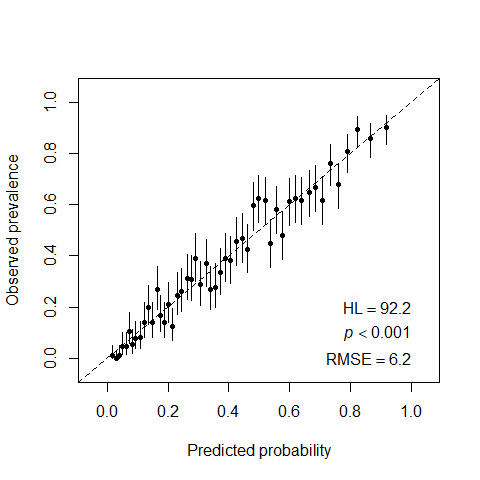Figure 1: Visual test of goodness-of-fit of a PS model and Hosmer-Lemeshow test.

Ideally, all the points should fall on the diagonal line. In our example, as the Hosmer and Lemeshow’s test shows, the logistic regression model with all the main effects does not fit the data well ($$pvalue<0.001$$), although visually the estimates fluctuate around the reference line.

For comparison, if we omit all the covariates that are insignificant at the 0.05 level from the original model, the new model perfectly fits the data:

ind <- ps.stab.ATE$summary.glm$coefficients[,4]<0.05
ps.stab.ATE.2 <- get.weights(df=rhc, A="RHC", C=covs.names[ind], wtype = "stab.ATE")
gof <- HLfit(obs=rhc$RHC, pred=ps.stab.ATE.2$ps, n.bins=50, bin.method = "quantiles", plot.bin.size=FALSE)
Arguments fixed.bin.size, min.bin.size and min.prob.interval are ignored by this bin.method.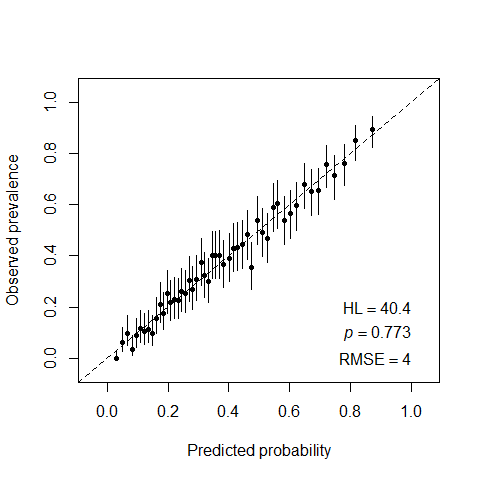Figure 2: Checking goodness-of-fit of a PS model with selection of significant covariates.

However, we notice that:

1. Although the omission of all insignificant covariates resulted in a better fit, using this selective model for weighting can leave the excluded covariates imbalanced, and moreover this might even distort the initially existing balance in them, as we can see further on Figure 5 which corresponds to the PS model with only significant covariates. The GOF criterion optimizes the loss function which does not necessarily goes along with balancing of important confounders.

2. Another potential “danger” of keeping only strong predictors of the treatment assingment in the PS model is an issue of possible bias amplification - see, for example, Ding, VanderWeele, and Robins (2017).

In summary, a good PS model cannot be built based only on the covariates-“treatment assignment” relationship. It should incorporate extra knowledge on the covariates-outcome relationship and other aspects of the data generating processes.

In what follows, we will proceed with the PS model that includes all the available covariates.

### Checking overlap

In order to see whether there is a non-overlap problem, we compare the distributions of estimated propensity scores in two treatment groups:

rhc.ps <- rhc %>% mutate(ps = ps.stab.ATE$ps, stab.ATE.w = ps.stab.ATE$w)
ggplot(rhc.ps, aes(x = ps, fill = trt, color=trt)) +  geom_density(alpha = 0.5) + scale_fill_npg() + scale_color_npg()+ theme(plot.title = element_text(hjust = 0.5), legend.position = c(0.8, 0.8),
legend.background=element_rect(fill="transparent"))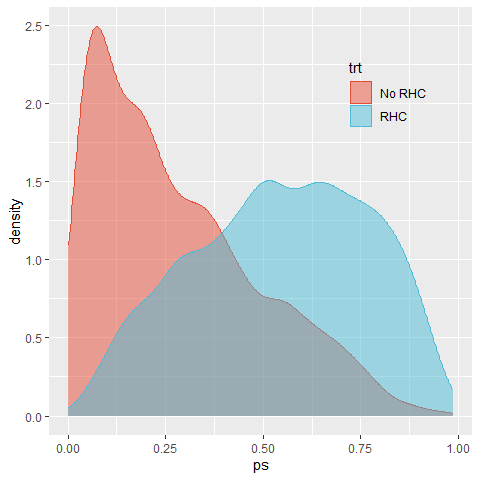Figure 3: Checking overlap in PS distributions.

The plot reveals that the majority of patients who actually did not go through RHC procedure, had low (<0.5) propensities to get it. In general, there is no clear violation of overlap, and both groups appear near both edges of the support. However, this plot is not able to identify the strata responsible for the most extreme propensities. We will address this question below by comparing between the overlap population and the original population mixes.

### Estimation of weights and balance diagnostics

In order to evaluate the attained mean covariate balance after weighting we will look at the absolute standardized differences, ASD (Li, Thomas, and Li (2018), Li, Morgan, and Zaslavsky (2018), Mao, Li, and Greene (2019)). For continuous covariates, we will also inspect the weighted marginal distributions.

We will compare already estimated stab.ATE weights with the overlap weights, estimated by running:

ps.overlap <- get.weights(df=rhc, A="RHC", C=covs.names, wtype = "overlap")
rhc.ps <- rhc.ps %>%   mutate(overlap.w = ps.overlap$w) A convenient way to summarize all the ASD of three weights (stab.ATE, overlap and original unadjusted population) is the love.plot from the cobalt package (Greifer (2020)): #fig.asp=1.1, covs <- subset(rhc.ps, select = covs.names) suppressWarnings(love.plot(RHC ~ covs, data=rhc.ps, weights = list(overlap=rhc.ps$overlap.w, stab.ATE=rhc.ps$stab.ATE.w), threshold = .1, abs=TRUE, binary = "std", stars="raw", s.d.denom="pooled", var.order = "unadjusted", title = "Comparing balancing weights") + scale_fill_npg() + scale_color_npg())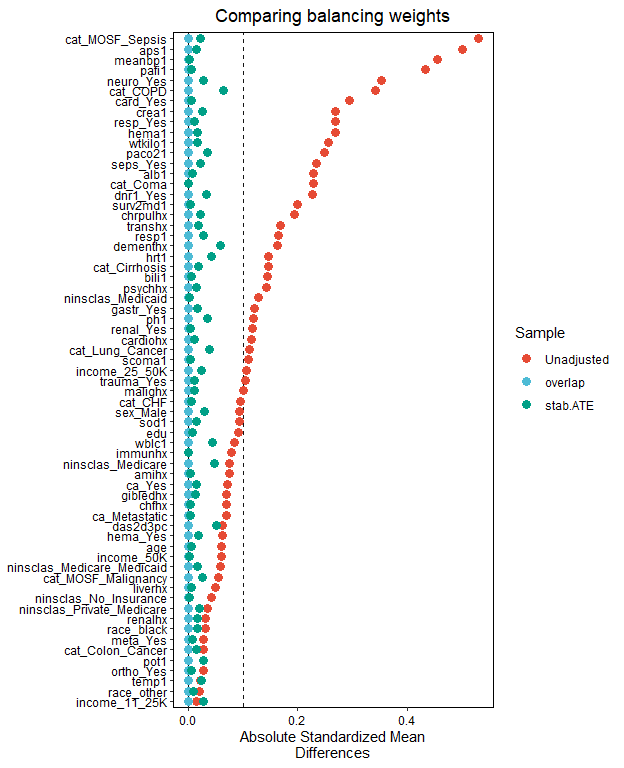Figure 4: Testing covariate balance of the PS model with all covariates. We follow the established convention that “a standardised difference of less than 0.1 can be considered as adequate balance” (Granger et al. (2020)). The stabilized ATE weighting resulted in good average balance, and overlap weights estimated from a logistic regression, as expected, yielded exact average balance (Li, Morgan, and Zaslavsky (2018)). For comparison, we inspect the balance attained by a “better-fitted” PS model which omitted all insignificant covariates: ps.overlap.2 <- get.weights(df=rhc, A="RHC", C=covs.names[ind], wtype = "overlap") rhc.ps <- rhc.ps %>% mutate(ps.2 = ps.stab.ATE.2$ps, stab.ATE.w.2 = ps.stab.ATE.2$w, overlap.w.2 = ps.overlap.2$w)
covs <- subset(rhc.ps, select = covs.names)
suppressWarnings(love.plot(RHC ~ covs, data=rhc.ps,
weights = list(overlap=rhc.ps$overlap.w.2, stab.ATE=rhc.ps$stab.ATE.w.2),
threshold = .1, abs=TRUE, binary = "std", stars="raw", s.d.denom="pooled",
title = "Comparing balancing weights") + scale_fill_npg() + scale_color_npg())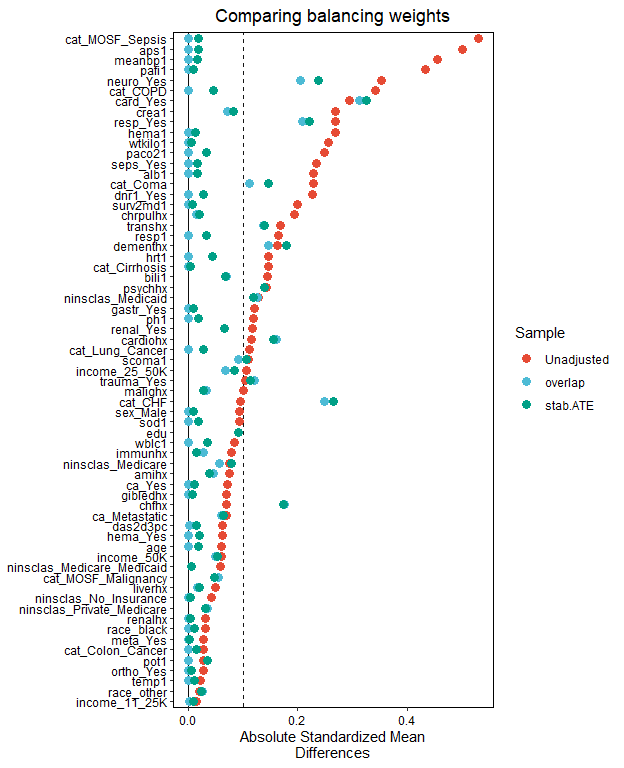Figure 5: Testing covariate balance of the PS model with selection of significant covariates.

Our conclusion is that the exclusion of insignificant variables from the PS model might result in disturbing the previously existing balance in some of those variables, without necessarily improving the balance in included covariates.

As an illustration of further balance diagnostics in continuous covariates, we chose two continuous variables with the least balance in the original population, and inspected how the weights performed in balancing the whole distribution.

For the APACHE score (aps1 variable):

rhc.ps$stab.ATE.w.1 <- rhc.ps$overlap.w.1<- rep(NA, nrow(rhc.ps))
rhc.ps$stab.ATE.w.1[rhc.ps$trt=="RHC"] <-
rhc.ps$stab.ATE.w[rhc.ps$trt=="RHC"]/sum(rhc.ps$stab.ATE.w[rhc.ps$trt=="RHC"])
rhc.ps$stab.ATE.w.1[rhc.ps$trt=="No RHC"] <-
rhc.ps$stab.ATE.w[rhc.ps$trt=="No RHC"]/sum(rhc.ps$stab.ATE.w[rhc.ps$trt=="No RHC"])
rhc.ps$overlap.w.1[rhc.ps$trt=="RHC"] <-
rhc.ps$overlap.w[rhc.ps$trt=="RHC"]/sum(rhc.ps$overlap.w[rhc.ps$trt=="RHC"])
rhc.ps$overlap.w.1[rhc.ps$trt=="No RHC"] <-
rhc.ps$overlap.w[rhc.ps$trt=="No RHC"]/sum(rhc.ps$overlap.w[rhc.ps$trt=="No RHC"])
ggplot(rhc.ps, aes(x = aps1, fill = trt, color=trt)) + ggtitle("A")+
geom_density(alpha = 0.5) + scale_fill_npg() + scale_color_npg()+
theme(plot.title = element_text(hjust = 0.5), legend.position = c(0.7, 0.7),
legend.background=element_rect(fill="transparent"))
ggplot(rhc.ps, aes(x = aps1, fill = trt, color=trt, weight=stab.ATE.w.1)) + ggtitle("B")+ geom_density(alpha = 0.5) + scale_fill_npg() + scale_color_npg()+
theme(plot.title = element_text(hjust = 0.5), legend.position = c(0.7, 0.7),
legend.background=element_rect(fill="transparent"))
ggplot(rhc.ps, aes(x = aps1, fill = trt, color=trt, weight=overlap.w.1)) + ggtitle("C")+  geom_density(alpha = 0.5) + scale_fill_npg() + scale_color_npg()+
theme(plot.title = element_text(hjust = 0.5), legend.position = c(0.7, 0.7),
legend.background=element_rect(fill="transparent"))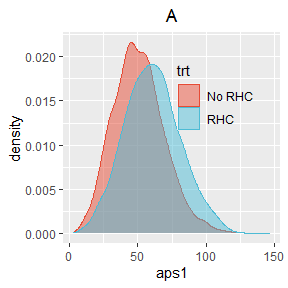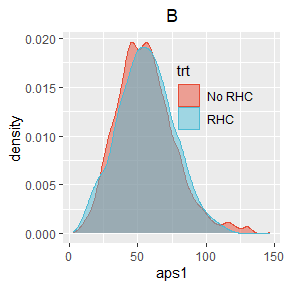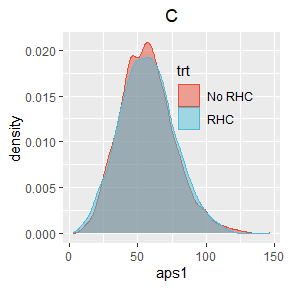Figure 6: Comparing distributions of aps1 across two treatment arms for: (A) unadjusted, (B) stab.ATE, (C) overlap weights

For the mean blood pressure (meanbp1 variable):

ggplot(rhc.ps, aes(x = meanbp1, fill = trt, color=trt)) + ggtitle("A")+
geom_density(alpha = 0.5) + scale_fill_npg() + scale_color_npg()+
theme(plot.title = element_text(hjust = 0.5), legend.position = c(0.7, 0.7),
legend.background=element_rect(fill="transparent"))
ggplot(rhc.ps, aes(x = meanbp1, fill = trt, color=trt, weight=stab.ATE.w.1)) + ggtitle("B")+ geom_density(alpha = 0.5) + scale_fill_npg() + scale_color_npg()+
theme(plot.title = element_text(hjust = 0.5), legend.position = c(0.7, 0.7),
legend.background=element_rect(fill="transparent"))
ggplot(rhc.ps, aes(x = meanbp1, fill = trt, color=trt, weight=overlap.w.1)) + ggtitle("C")+  geom_density(alpha = 0.5) + scale_fill_npg() + scale_color_npg()+
theme(plot.title = element_text(hjust = 0.5), legend.position = c(0.7, 0.7),
legend.background=element_rect(fill="transparent"))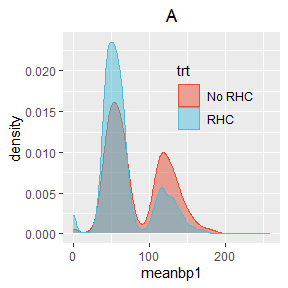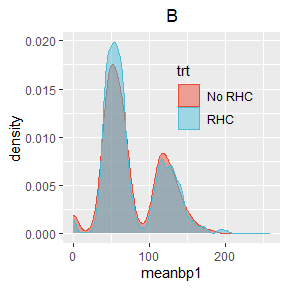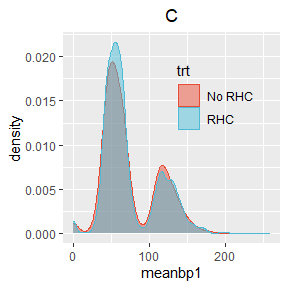Figure 7: Comparing distributions of meanbp1 across two treatment arms for: (A) unadjusted, (B) stab.ATE, (C) overlap weights

For both variables, the balance achieved by stab.ATE and overlap weights looks pretty adequate.

### Characterizing the target population

It is very important to describe the baseline characteristics of the target population for which we are going to estimate the ATE, since it can help us to understand whether a pseudo-population created by weighting is clinically relevant and which randomized trial is best emulated by the chosen weights (Thomas, Li, and Pencina (2020)). In Table 1 we summarize the marginal covariate distributions for three populations:

1. original (unweighted) population,
2. the population created by stab.ATE weights and
3. the overlap population
d <- rhc.ps[, covs.names]
add.names <- function(x, a) { paste(x,a, sep="")}
# original population:
descr.orig <- round( cbind(
apply(d, 2, wtd.mean, weights=rep(1, nrow(d))),
sqrt(apply(d, 2, wtd.var, weights=rep(1, nrow(d)))),
t(apply(d, 2, wtd.quantile, weights=rep(1, nrow(d)), probs=c(.25, .5, .75)))
), dig=2)
descr.ATE <- round( cbind(
apply(d, 2, wtd.mean, weights=rhc.ps$stab.ATE.w), sqrt(apply(d, 2, wtd.var, weights=rhc.ps$stab.ATE.w)),
t(apply(d, 2, wtd.quantile, weights=rhc.ps$stab.ATE.w, probs=c(.25, .5, .75))) ), dig=2) descr.overlap <- round( cbind( apply(d, 2, wtd.mean, weights=rhc.ps$overlap.w),
sqrt(apply(d, 2, wtd.var, weights=rhc.ps$overlap.w)), t(apply(d, 2, wtd.quantile, weights=rhc.ps$overlap.w, probs=c(.25, .5, .75)))
), dig=2)
df3 <- data.frame(cbind(descr.orig, descr.ATE, descr.overlap))
row.names(df3) <- covs.names
names(df3) <- rep( c("mean", "sd", "q1", "med", "q3"), times=3)

kable(df3, caption="Comparing marginal distributions of covariates for three populations. q1, med and q3 correspond to 1st, 2nd and 3rd quartiles of covariates distributions for: (1) the original  population; (2) stab.ATE population; (3) overlap population.") 
Table 1: Comparing marginal distributions of covariates for three populations. q1, med and q3 correspond to 1st, 2nd and 3rd quartiles of covariates distributions for: (1) the original population; (2) stab.ATE population; (3) overlap population.
mean sd q1 med q3 mean sd q1 med q3 mean sd q1 med q3
age 61.38 16.68 50.15 64.05 73.94 61.23 16.49 50.43 63.89 73.62 60.93 16.45 49.85 63.65 73.29
sex_Male 0.56 0.50 0.00 1.00 1.00 0.56 0.50 0.00 1.00 1.00 0.57 0.50 0.00 1.00 1.00
edu 11.68 3.15 10.00 12.00 13.00 11.70 3.07 10.00 12.00 13.00 11.78 3.12 10.00 12.00 13.61
race_black 0.16 0.37 0.00 0.00 0.00 0.16 0.37 0.00 0.00 0.00 0.16 0.36 0.00 0.00 0.00
race_other 0.06 0.24 0.00 0.00 0.00 0.06 0.23 0.00 0.00 0.00 0.06 0.24 0.00 0.00 0.00
income_11_25K 0.20 0.40 0.00 0.00 0.00 0.20 0.40 0.00 0.00 0.00 0.21 0.41 0.00 0.00 0.00
income_25_50K 0.16 0.36 0.00 0.00 0.00 0.15 0.36 0.00 0.00 0.00 0.16 0.37 0.00 0.00 0.00
income_50K 0.08 0.27 0.00 0.00 0.00 0.08 0.27 0.00 0.00 0.00 0.08 0.28 0.00 0.00 0.00
ninsclas_Private_Medicare 0.22 0.41 0.00 0.00 0.00 0.22 0.41 0.00 0.00 0.00 0.23 0.42 0.00 0.00 0.00
ninsclas_Medicare 0.25 0.44 0.00 0.00 1.00 0.25 0.43 0.00 0.00 1.00 0.24 0.43 0.00 0.00 0.00
ninsclas_Medicare_Medicaid 0.07 0.25 0.00 0.00 0.00 0.06 0.24 0.00 0.00 0.00 0.06 0.24 0.00 0.00 0.00
ninsclas_Medicaid 0.11 0.32 0.00 0.00 0.00 0.12 0.32 0.00 0.00 0.00 0.10 0.30 0.00 0.00 0.00
ninsclas_No_Insurance 0.06 0.23 0.00 0.00 0.00 0.06 0.23 0.00 0.00 0.00 0.06 0.23 0.00 0.00 0.00
cat_CHF 0.08 0.27 0.00 0.00 0.00 0.08 0.28 0.00 0.00 0.00 0.10 0.30 0.00 0.00 0.00
cat_Cirrhosis 0.05 0.21 0.00 0.00 0.00 0.04 0.20 0.00 0.00 0.00 0.04 0.19 0.00 0.00 0.00
cat_Colon_Cancer 0.00 0.04 0.00 0.00 0.00 0.00 0.04 0.00 0.00 0.00 0.00 0.03 0.00 0.00 0.00
cat_Coma 0.09 0.29 0.00 0.00 0.00 0.09 0.29 0.00 0.00 0.00 0.07 0.25 0.00 0.00 0.00
cat_COPD 0.08 0.27 0.00 0.00 0.00 0.07 0.26 0.00 0.00 0.00 0.04 0.20 0.00 0.00 0.00
cat_Lung_Cancer 0.01 0.10 0.00 0.00 0.00 0.01 0.09 0.00 0.00 0.00 0.00 0.07 0.00 0.00 0.00
cat_MOSF_Malignancy 0.11 0.31 0.00 0.00 0.00 0.11 0.32 0.00 0.00 0.00 0.12 0.32 0.00 0.00 0.00
cat_MOSF_Sepsis 0.36 0.48 0.00 0.00 1.00 0.37 0.48 0.00 0.00 1.00 0.41 0.49 0.00 0.00 1.00
dnr1_Yes 0.11 0.32 0.00 0.00 0.00 0.11 0.31 0.00 0.00 0.00 0.09 0.29 0.00 0.00 0.00
wtkilo1 67.82 29.06 56.30 70.00 83.70 68.43 28.94 56.74 70.00 84.00 69.75 27.25 58.30 71.00 84.50
hrt1 115.17 41.25 97.00 124.00 141.00 114.62 42.36 95.00 124.00 142.00 116.52 41.53 102.71 124.00 144.00
meanbp1 78.52 38.05 50.00 63.00 115.00 77.32 38.86 49.00 63.00 114.00 74.13 36.17 49.00 61.00 110.00
resp1 28.09 14.08 14.00 30.00 38.00 28.27 14.27 15.00 30.00 38.00 28.19 14.05 16.00 30.00 38.00
temp1 37.62 1.77 36.09 38.09 39.00 37.58 1.82 36.09 38.09 39.00 37.63 1.81 36.09 38.20 39.00
card_Yes 0.34 0.47 0.00 0.00 1.00 0.35 0.48 0.00 0.00 1.00 0.38 0.48 0.00 0.00 1.00
gastr_Yes 0.16 0.37 0.00 0.00 0.00 0.17 0.37 0.00 0.00 0.00 0.18 0.38 0.00 0.00 0.00
hema_Yes 0.06 0.24 0.00 0.00 0.00 0.06 0.24 0.00 0.00 0.00 0.06 0.24 0.00 0.00 0.00
meta_Yes 0.05 0.21 0.00 0.00 0.00 0.05 0.21 0.00 0.00 0.00 0.05 0.21 0.00 0.00 0.00
neuro_Yes 0.12 0.33 0.00 0.00 0.00 0.12 0.32 0.00 0.00 0.00 0.08 0.27 0.00 0.00 0.00
ortho_Yes 0.00 0.03 0.00 0.00 0.00 0.00 0.03 0.00 0.00 0.00 0.00 0.03 0.00 0.00 0.00
renal_Yes 0.05 0.22 0.00 0.00 0.00 0.06 0.23 0.00 0.00 0.00 0.06 0.23 0.00 0.00 0.00
resp_Yes 0.37 0.48 0.00 0.00 1.00 0.37 0.48 0.00 0.00 1.00 0.34 0.48 0.00 0.00 1.00
seps_Yes 0.18 0.38 0.00 0.00 0.00 0.18 0.39 0.00 0.00 0.00 0.21 0.41 0.00 0.00 0.00
trauma_Yes 0.01 0.09 0.00 0.00 0.00 0.01 0.09 0.00 0.00 0.00 0.01 0.09 0.00 0.00 0.00
ca_Yes 0.17 0.38 0.00 0.00 0.00 0.17 0.38 0.00 0.00 0.00 0.17 0.38 0.00 0.00 0.00
ca_Metastatic 0.07 0.25 0.00 0.00 0.00 0.07 0.25 0.00 0.00 0.00 0.06 0.24 0.00 0.00 0.00
amihx 0.03 0.18 0.00 0.00 0.00 0.03 0.17 0.00 0.00 0.00 0.03 0.18 0.00 0.00 0.00
cardiohx 0.18 0.38 0.00 0.00 0.00 0.19 0.39 0.00 0.00 0.00 0.20 0.40 0.00 0.00 0.00
chfhx 0.18 0.38 0.00 0.00 0.00 0.18 0.39 0.00 0.00 0.00 0.20 0.40 0.00 0.00 0.00
chrpulhx 0.19 0.39 0.00 0.00 0.00 0.18 0.39 0.00 0.00 0.00 0.16 0.37 0.00 0.00 0.00
dementhx 0.10 0.30 0.00 0.00 0.00 0.09 0.29 0.00 0.00 0.00 0.08 0.27 0.00 0.00 0.00
gibledhx 0.03 0.18 0.00 0.00 0.00 0.03 0.17 0.00 0.00 0.00 0.03 0.17 0.00 0.00 0.00
immunhx 0.27 0.44 0.00 0.00 1.00 0.27 0.45 0.00 0.00 1.00 0.28 0.45 0.00 0.00 1.00
liverhx 0.07 0.26 0.00 0.00 0.00 0.07 0.25 0.00 0.00 0.00 0.07 0.25 0.00 0.00 0.00
malighx 0.23 0.42 0.00 0.00 0.00 0.23 0.42 0.00 0.00 0.00 0.23 0.42 0.00 0.00 0.00
psychhx 0.07 0.25 0.00 0.00 0.00 0.06 0.24 0.00 0.00 0.00 0.06 0.23 0.00 0.00 0.00
renalhx 0.04 0.21 0.00 0.00 0.00 0.05 0.21 0.00 0.00 0.00 0.05 0.22 0.00 0.00 0.00
transhx 0.12 0.32 0.00 0.00 0.00 0.12 0.32 0.00 0.00 0.00 0.12 0.33 0.00 0.00 0.00
aps1 54.67 19.96 41.00 54.00 67.00 55.83 21.00 41.00 55.00 69.00 56.93 19.85 43.00 56.00 69.13
das2d3pc 20.50 5.32 16.06 19.75 23.44 20.49 5.29 16.06 19.66 23.57 20.55 5.24 16.39 19.81 23.46
scoma1 21.00 30.27 0.00 0.00 41.00 20.86 29.78 0.00 0.00 41.00 19.38 28.94 0.00 0.00 37.00
surv2md1 0.59 0.20 0.47 0.63 0.74 0.58 0.20 0.46 0.62 0.74 0.58 0.20 0.46 0.62 0.74
alb1 3.09 0.78 2.60 3.50 3.50 3.09 0.94 2.60 3.50 3.50 3.05 0.89 2.50 3.50 3.50
bili1 2.27 4.80 0.80 1.01 1.40 2.37 4.98 0.80 1.01 1.50 2.50 5.24 0.90 1.01 1.50
crea1 2.13 2.05 1.00 1.50 2.40 2.16 2.12 1.00 1.50 2.40 2.26 2.15 1.10 1.60 2.60
hema1 31.87 8.36 26.10 30.00 36.30 31.48 8.35 26.00 29.50 35.59 31.01 7.90 26.00 29.10 34.50
paco21 38.75 13.18 31.00 37.00 42.00 38.79 13.29 31.00 37.00 42.00 37.74 11.57 31.00 36.00 41.00
pafi1 222.26 114.96 133.31 202.50 316.62 219.28 115.20 130.59 199.99 312.50 211.86 108.90 126.66 190.47 296.30
ph1 7.39 0.11 7.34 7.40 7.46 7.39 0.12 7.33 7.40 7.46 7.39 0.11 7.34 7.40 7.46
pot1 4.07 1.03 3.40 3.80 4.60 4.06 1.05 3.40 3.80 4.60 4.05 1.02 3.40 3.80 4.50
sod1 136.77 7.65 132.00 136.00 142.00 136.78 7.53 132.00 136.00 142.00 136.61 7.57 132.00 136.00 142.00
wblc1 15.65 11.87 8.40 14.10 20.07 16.19 13.55 8.60 14.10 20.50 15.83 12.51 8.30 14.20 20.41
#%>% kable_styling(bootstrap_options = c("striped", "hover", "condensed", "responsive"), full_width = FALSE) %>%
#   add_header_above(c("covariate "= 1, "original population" = 5, "stab.ATE population" = 5, "overlap population"=5)) %>%
# column_spec(2, background = "lightgrey") %>%
#  column_spec(7, background = "lightgrey") %>%
#  column_spec(12, background = "lightgrey") %>%
#    scroll_box(width = "100%", height = "500px")

There is not observed any significant difference between the target populations (i.e., ATE and overlap populations), both of which are similar to the original population.
The stab.ATE weighting is expected to produce a population which is similar to the original population on average, with respect to covariates included in the PS model. In stab.ATE weighting no observation is excluded from the analysis, i.e. no weights are close to zero. But there could be possibly extremely influential observations with relatively high weights. Those are the observations that are rarely represented in their assigned treatment group. Figure 8 shows the distributions of stab.ATE weights.

ggplot(rhc.ps, aes(x = stab.ATE.w, fill = trt, color=trt)) +
geom_histogram(alpha = 0.4, bins=30) + scale_fill_npg() + scale_color_npg()+ theme(plot.title = element_text(hjust = 0.5), legend.position = c(0.8, 0.8),
legend.background=element_rect(fill="transparent")) + ggtitle("Distribution of stab.ATE weights")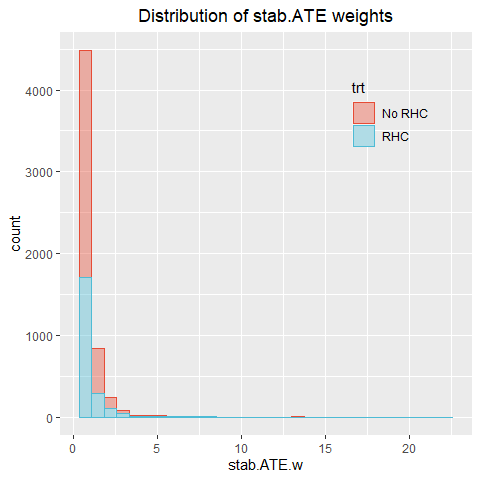Figure 8: Distribution of stab.ATE weights.

Table 2 lists observations with stab.ATE weights greater than 10, together with their overlap weights. Notice that these observations have the highest overlap weights as well, but since overlap weights are bounded between 0 and 1, their influence in overlap weighting will be diminished.

kable(rhc.ps[rhc.ps$stab.ATE.w>10, c("trt", "stab.ATE.w", "overlap.w", "ps")], caption="The list of most influential observations in the stab.ATE weighting.") Table 2: The list of most influential observations in the stab.ATE weighting. trt stab.ATE.w overlap.w ps 505 No RHC 17.82322 0.9652538 0.9652538 1000 No RHC 14.74340 0.9579956 0.9579956 2174 No RHC 13.58583 0.9544166 0.9544166 3145 No RHC 13.41335 0.9538304 0.9538304 3216 No RHC 15.75661 0.9606966 0.9606966 4007 No RHC 19.30655 0.9679234 0.9679234 4131 RHC 10.45646 0.9635908 0.0364092 4890 RHC 21.90397 0.9826191 0.0173809 In the overlap weighting, the “influential” observations are defined in a different way from the stab.ATE weighting. If a person with covariates $$C=c$$ has high probability of being assigned to his actual treatment group, he would have low probability of appearing in the opposite group, meaning that if he would appear in the opposite group he would be highly influential. That is why, this “potentially influential” person will get a near 0 overlap weight in order to reduce his potential influence. Those potentially influential observations are different from those actually influential observations in stab.ATE weighting. Figure 9 shows the distributions of overlap weights. ggplot(rhc.ps, aes(x = overlap.w, fill = trt, color=trt)) + geom_histogram(alpha = 0.4, bins=30) + scale_fill_npg() + scale_color_npg()+ theme(plot.title = element_text(hjust = 0.5), legend.position = c(0.8, 0.8), legend.background=element_rect(fill="transparent")) + ggtitle("Distribution of overlap weights")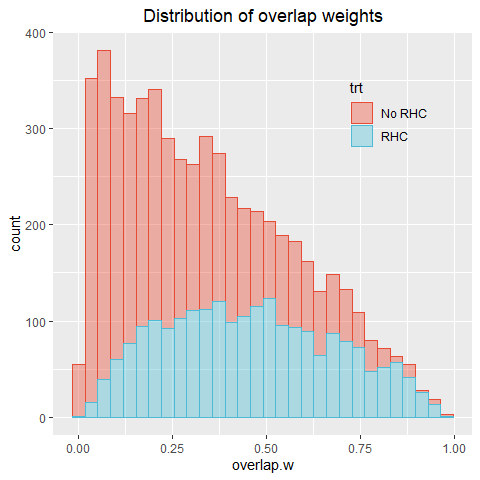Figure 9: Distribution of overlap weights. We will base our final estimates on stabilized ATE weights. ## Estimating the effect of RHC on the length-of-stay ### Graphical test of a proportional hazards assumption We estimate all the parameters using both nonparametric and Cox-based approaches: # overlap weights========================: # Nonparametric estimation: res.overlap <- fit.nonpar(df=rhc, X="Time", E="E", A="RHC", C=covs.names, wtype="overlap", cens=0, conf.level=0.95, bs=TRUE, nbs.rep=50, seed=17, parallel = FALSE) # The small number of bootstrap replications (nbs.rep=80) was chosen for illustration purposes. # Cox-based estimation: res.cox.overlap <- fit.cox(df=rhc, X="Time", E="E", A="RHC", C=covs.names, wtype="overlap", cens=0, conf.level=0.95, bs=TRUE, nbs.rep=50, seed=17, parallel = FALSE) # stab.ATE weights==========================: # Nonparametric estimation: res.stab.ATE <- fit.nonpar(df=rhc, X="Time", E="E", A="RHC", C=covs.names, wtype="stab.ATE", cens=0, conf.level=0.95, bs=TRUE, nbs.rep=50, seed=17, parallel = FALSE) # Cox-based estimation: res.cox.stab.ATE <- fit.cox(df=rhc, X="Time", E="E", A="RHC", C=covs.names, wtype="stab.ATE", cens=0, conf.level=0.95, bs=TRUE, nbs.rep=50, seed=17, parallel = FALSE) # unadjusted analysis========================: # Nonparametric estimation: res.un <- fit.nonpar(df=rhc, X="Time", E="E", A="RHC", C=covs.names, wtype="unadj", cens=0, conf.level=0.95, bs=TRUE, nbs.rep=50, seed=17, parallel = FALSE) # Cox-based estimation: res.cox.un <- fit.cox(df=rhc, X="Time", E="E", A="RHC", C=covs.names, wtype="unadj", cens=0, conf.level=0.95, bs=TRUE, nbs.rep=50, seed=17, parallel = FALSE) We aggregate log-ratios of cumulative hazards from nonparametric and Cox-based estimation into one data frame for each type of weights: get.logCumHR <- function(res, res.cox) { df1 <- rbind( data.frame(time=res$time, Outcome=1,
CumHazR=res$trt.eff[[paste("Ev=", 1, sep="")]]$log.CumHazR,
CIL.CumHazR=res$trt.eff[[paste("Ev=", 1, sep="")]]$log.CumHazR.CI.L,
CIU.CumHazR=res$trt.eff[[paste("Ev=", 1, sep="")]]$log.CumHazR.CI.U),
data.frame(time=res$time, Outcome=2, CumHazR=res$trt.eff[[paste("Ev=", 2, sep="")]]$log.CumHazR, CIL.CumHazR=res$trt.eff[[paste("Ev=", 2, sep="")]]$log.CumHazR.CI.L, CIU.CumHazR=res$trt.eff[[paste("Ev=", 2, sep="")]]$log.CumHazR.CI.U)) df2 <- data.frame(time=c(min(res.cox$time), max(res.cox$time)), Outcome=rep(3, 2), CumHazR=rep(res.cox$trt.eff[[paste("Ev=", 1, sep="")]]$log.CumHazR, 2), CIL.CumHazR=rep(NA, 2), CIU.CumHazR=rep(NA, 2)) df3 <- data.frame(time=c(min(res.cox$time), max(res.cox$time)), Outcome=rep(4, 2), CumHazR=rep(res.cox$trt.eff[[paste("Ev=", 2, sep="")]]$log.CumHazR, 2), CIL.CumHazR=rep(NA, 2), CIU.CumHazR=rep(NA, 2)) df <- rbind(df1, df2, df3) df$Outcome <- factor(df$Outcome) levels(df$Outcome) <- c("Discharge-nonpar", "Death-nonpar",
paste("Discharge-Cox:", round(res.cox$trt.eff[[paste("Ev=", 1, sep="")]]$log.CumHazR,dig=2),
", 95% CI=[",
round(res.cox$trt.eff[[paste("Ev=", 1, sep="")]]$log.CumHazR.CI.L,dig=2),
",",
round(res.cox$trt.eff[[paste("Ev=", 1, sep="")]]$log.CumHazR.CI.U,dig=2),
"]", sep=""),
paste("Death-Cox:", round(res.cox$trt.eff[[paste("Ev=", 2, sep="")]]$log.CumHazR,dig=2), ", 95% CI=[",
round(res.cox$trt.eff[[paste("Ev=", 2, sep="")]]$log.CumHazR.CI.L,dig=2),
",",
round(res.cox$trt.eff[[paste("Ev=", 2, sep="")]]$log.CumHazR.CI.U,dig=2),
"]", sep=""))
return(df)
}
logCumHR.overlap <- get.logCumHR(res.overlap, res.cox.overlap)
logCumHR.stab.ATE <- get.logCumHR(res.stab.ATE, res.cox.stab.ATE)
logCumHR.unadj <- get.logCumHR(res.un, res.cox.un)

A standard way to test a proportional hazards assumption is by plotting the log-ratio, $$\log \frac{H_k^1(t)}{H_k^0(t)}$$, $$k=1,2$$, versus $$t$$, with $$H_k^a(t)$$, $$a=0,1$$ estimated nonparametrically. Under the Cox proportional hazards model, this log-ratio equals $$\beta$$ that does not depend on time:

plot.logCumHazR <- function(df, title)
{
p <- ggplot(df, aes(x=time, y=CumHazR, color=Outcome, fill=Outcome, shape=Outcome)) + ggtitle(title) +
geom_step(size=1.1) +
geom_ribbon(aes(ymin=CIL.CumHazR, ymax=CIU.CumHazR), alpha=0.2, stat="stepribbon") +
scale_fill_npg() + scale_color_npg()
p <- p + xlab("time from admission to ICU (days)") + ylab("log.CumHR(t)") +
theme(axis.text.x = element_text(face="bold", angle=45),
axis.text.y = element_text(face="bold"), plot.title = element_text(hjust = 0.5))+
theme(legend.position = c(0.5, 0.3),
legend.background=element_rect(fill="transparent"),
panel.grid.minor = element_line(size = .5,colour = "gray92"),
panel.grid.major = element_line(size = .5,colour = "#C0C0C0")) +
geom_vline(xintercept=30, linetype="dashed")
p
}

plot.logCumHazR(df=logCumHR.overlap, title="Overlap weights")
plot.logCumHazR(df=logCumHR.stab.ATE, title="Stabilized ATE weights")
plot.logCumHazR(df=logCumHR.unadj, title="Unadjusted")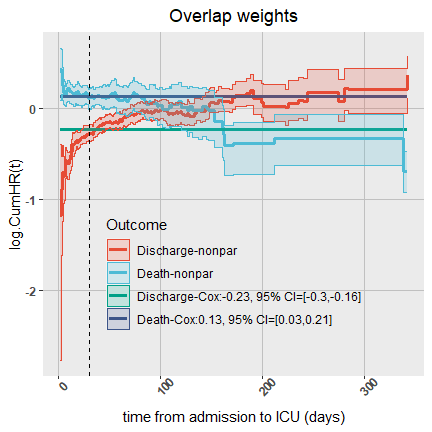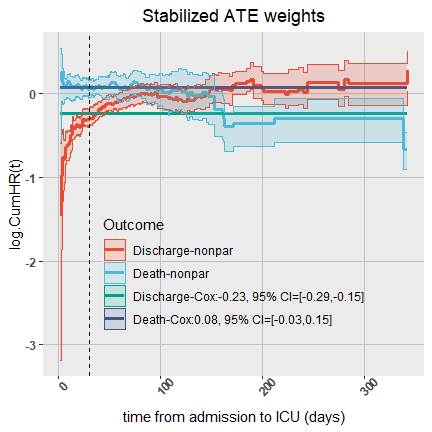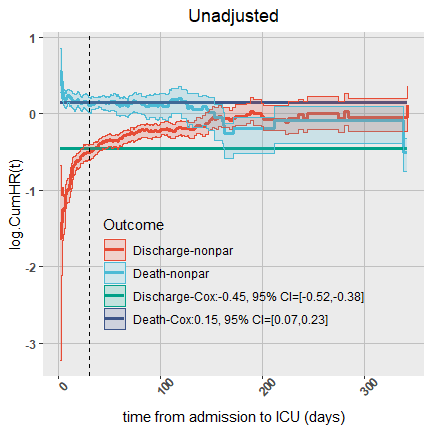Figure 10: log(Cum.HR(t)) of RHC vs No-RHC for discharge and in-hospital death.

We will focus on the stabilized ATE weighting analysis.

### Estimating cause-specific hazards, risk and RMT

#### Comparing cause-specific hazards over 3 populations

We aggregate hazards obtained from three weighting schemes, overlap, stab.ATE and unadjusted, into one data frame and compare the absolute cumulative hazards for three populations:

get.CumHaz <- function(res1, res2, res3, Ev)
{
df <- rbind( data.frame(time=res1$time, population=1, TRT=1, CumHaz=res1$trt.1[[paste("Ev=", Ev, sep="")]]$CumHaz, CIL.CumHaz=res1$trt.1[[paste("Ev=", Ev, sep="")]]$CumHaz.CI.L, CIU.CumHaz=res1$trt.1[[paste("Ev=", Ev, sep="")]]$CumHaz.CI.U), data.frame(time=res2$time, population=2, TRT=1,
CumHaz=res2$trt.1[[paste("Ev=", Ev, sep="")]]$CumHaz,
CIL.CumHaz=res2$trt.1[[paste("Ev=", Ev, sep="")]]$CumHaz.CI.L,
CIU.CumHaz=res2$trt.1[[paste("Ev=", Ev, sep="")]]$CumHaz.CI.U),
data.frame(time=res3$time, population=3, TRT=1, CumHaz=res3$trt.1[[paste("Ev=", Ev, sep="")]]$CumHaz, CIL.CumHaz=res3$trt.1[[paste("Ev=", Ev, sep="")]]$CumHaz.CI.L, CIU.CumHaz=res3$trt.1[[paste("Ev=", Ev, sep="")]]$CumHaz.CI.U), data.frame(time=res1$time, population=1, TRT=0,
CumHaz=res1$trt.0[[paste("Ev=", Ev, sep="")]]$CumHaz,
CIL.CumHaz=res1$trt.0[[paste("Ev=", Ev, sep="")]]$CumHaz.CI.L,
CIU.CumHaz=res1$trt.0[[paste("Ev=", Ev, sep="")]]$CumHaz.CI.U),
data.frame(time=res2$time, population=2, TRT=0, CumHaz=res2$trt.0[[paste("Ev=", Ev, sep="")]]$CumHaz, CIL.CumHaz=res2$trt.0[[paste("Ev=", Ev, sep="")]]$CumHaz.CI.L, CIU.CumHaz=res2$trt.0[[paste("Ev=", Ev, sep="")]]$CumHaz.CI.U), data.frame(time=res3$time, population=3, TRT=0,
CumHaz=res3$trt.0[[paste("Ev=", Ev, sep="")]]$CumHaz,
CIL.CumHaz=res3$trt.0[[paste("Ev=", Ev, sep="")]]$CumHaz.CI.L,
CIU.CumHaz=res3$trt.0[[paste("Ev=", Ev, sep="")]]$CumHaz.CI.U)
)
df$population <- factor(df$population)
levels(df$population) <- c("overlap", "stab.ATE", "unadjusted") df } df.CumHaz <- list() E.set <- sort(unique(rhc$E))
E.set <- E.set[E.set!=0] # 0 is for censoring status
for (k in E.set)
df.CumHaz[[k]] <- get.CumHaz(res.overlap, res.stab.ATE, res.un, k)

plot.CumHaz <- function(df, title, ymax)
{
p <- ggplot(df, aes(x=time, y=CumHaz, color=population, fill=population, shape=population)) + ggtitle(title) +
geom_step(size=1.1) +
#     geom_ribbon(aes(ymin=CIL.CumHaz, ymax=CIU.CumHaz), alpha=0.2, stat="stepribbon") +
scale_fill_npg() + scale_color_npg()
p <- p + xlab("time from admission to ICU (days)") + ylab("Cumulative Hazard (t)") + ylim(0, ymax)+
theme(axis.text.x = element_text(face="bold", angle=45),
axis.text.y = element_text(face="bold"), plot.title = element_text(hjust = 0.5))+
theme(legend.position = c(0.7, 0.3),
legend.background=element_rect(fill="transparent"),
panel.grid.minor = element_line(size = .5,colour = "gray92"),
panel.grid.major = element_line(size = .5,colour = "#C0C0C0")) +
geom_vline(xintercept=30, linetype="dashed")
p
}

In the following plot we compare the absolute cumulative hazards across three populations:

plot.CumHaz(df=df.CumHaz[][df.CumHaz[]$TRT==1,], title="RHC: CumHaz of Discharge", ymax=6) plot.CumHaz(df=df.CumHaz[][df.CumHaz[]$TRT==0,], title="No-RHC: CumHaz of Discharge", ymax=6)
plot.CumHaz(df=df.CumHaz[][df.CumHaz[]$TRT==1,], title="RHC: CumHaz of Death", ymax=2.5) plot.CumHaz(df=df.CumHaz[][df.CumHaz[]$TRT==0,], title="No-RHC: CumHaz of Death", ymax=2.5)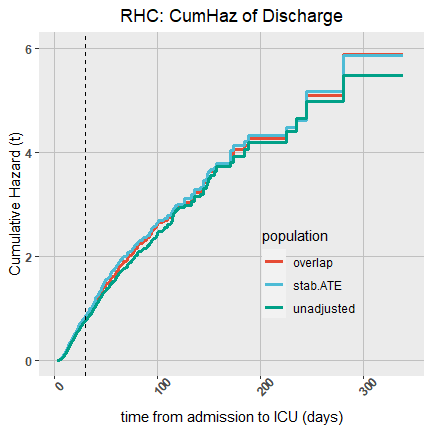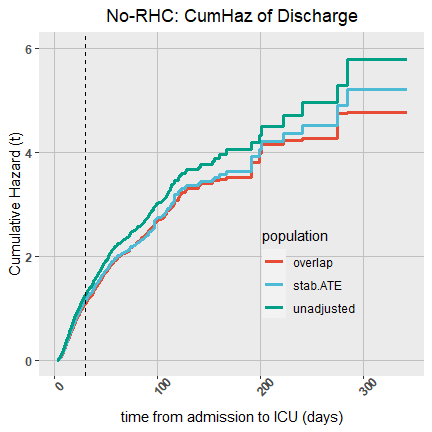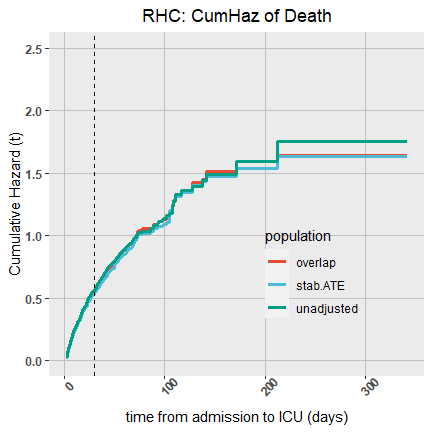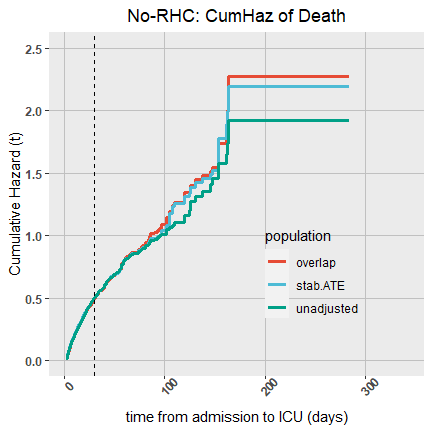Figure 11: Cumulative hazards for discharge and in-hospital death. Comparison of 3 target populations.

#### Cause-specific cumulative hazards for stabilized ATE weights

df <- rbind(summary(res.stab.ATE, event=1, estimand="CumHaz"),
summary(res.stab.ATE, event=2, estimand="CumHaz"))
df$Event_TRT <- factor(2*(df$Event==2) + 1*(df$TRT==0)) levels(df$Event_TRT) <- c("Discharge-RHC", "Discharge-No RHC",
"In-hospital death-RHC", "In-hospital death-No RHC")

ggplot(df, aes(x=time, y=CumHaz, color=Event_TRT, fill=Event_TRT,
shape=Event_TRT, linetype=Event_TRT)) + geom_step(linewidth=1.1) +
geom_ribbon(aes(ymin=CIL.CumHaz, ymax=CIU.CumHaz), alpha=0.2,
stat="stepribbon") + scale_color_brewer(palette = "Set1") +
scale_fill_brewer(palette = "Set1") + xlab("time from admission to ICU (days)") +
ylab("Cumulative hazard of event by time t") +
theme(legend.position = c(0.41, 0.83), legend.text=element_text(size=12),
legend.background=element_rect(fill="transparent"), legend.title=element_blank())+
geom_vline(xintercept=30, linetype="dashed")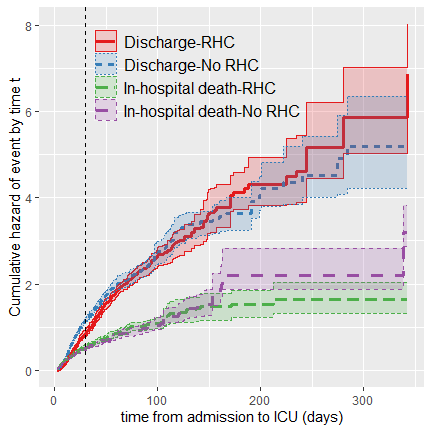Figure 12: Cause-specific cumulative hazards.

#### Risk curves or cumulative incidence functions (CIF) for stabilized ATE weights

df <- rbind(summary(res.stab.ATE, event=1, estimand="CIF"),
summary(res.stab.ATE, event=2, estimand="CIF"))
df$Event_TRT <- factor(2*(df$Event==2) + 1*(df$TRT==0)) levels(df$Event_TRT) <- c("Discharge-RHC", "Discharge-No RHC",
"In-hospital death-RHC", "In-hospital death-No RHC")

ggplot(df, aes(x=time, y=CIF, color=Event_TRT, fill=Event_TRT,
shape=Event_TRT, linetype=Event_TRT)) + geom_step(linewidth=1.1) + xlim(0, 200) +
geom_ribbon(aes(ymin=CIL.CIF, ymax=CIU.CIF), alpha=0.2,
stat="stepribbon") + scale_color_brewer(palette = "Set1") +
scale_fill_brewer(palette = "Set1") + xlab("time from admission to ICU (days)") +
ylab("Probability of event by time t") +
theme(legend.position = c(0.7, 0.25), legend.text=element_text(size=12),
legend.background=element_rect(fill="transparent"), legend.title=element_blank())+
geom_vline(xintercept=30, linetype="dashed")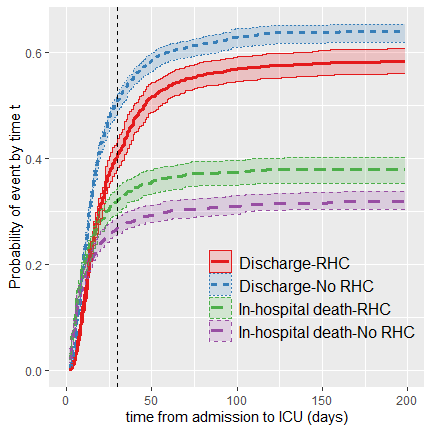Figure 13: Risk curves

#### RMT for stabilized ATE weights

An alternative way to summarize risk curves over time is to estimate the area under a risk curve. For a “good” outcome, e.g., discharge, the larger the area, the better the treatment. And, the opposite holds for a “bad” outcome, e.g. death, for which the smaller the area is, the better.

df <- rbind(summary(res.stab.ATE, event=1, estimand="RMT"),
summary(res.stab.ATE, event=2, estimand="RMT"))
df$Event_TRT <- factor(2*(df$Event==2) + 1*(df$TRT==0)) levels(df$Event_TRT) <- c("Discharge-RHC", "Discharge-No RHC",
"In-hospital death-RHC", "In-hospital death-No RHC")

ggplot(df, aes(x=time, y=RMT, color=Event_TRT, fill=Event_TRT,
shape=Event_TRT, linetype=Event_TRT)) + geom_line(linewidth=1.1) +
geom_ribbon(aes(ymin=CIL.RMT, ymax=CIU.RMT), alpha=0.2) +
scale_color_brewer(palette = "Set1") +
scale_fill_brewer(palette = "Set1") + xlab("time from admission to ICU (days)") +
ylab("average time lost due to death (gained by recovery)") +
theme(legend.position = c(0.35, 0.8), legend.text=element_text(size=12),
legend.background=element_rect(fill="transparent"), legend.title=element_blank())+
geom_vline(xintercept=30, linetype="dashed")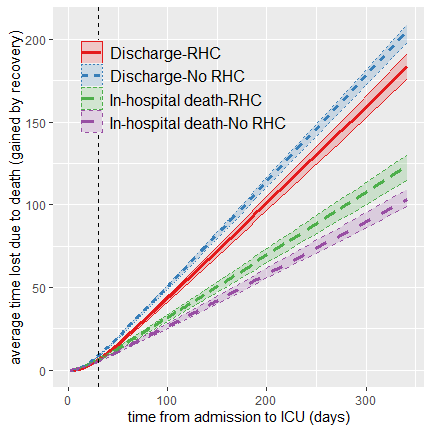Figure 14: Restricted-mean-time-lost/gained due to specific event.

### Estimating treatment effects for stabilized ATE weights

df <- rbind(summary(res.stab.ATE, event=1, estimand="RD"),
summary(res.stab.ATE, event=2, estimand="RD"))
df$Event <- as.factor(df$Event)
levels(df$Event) <- c("Discharge", "In-hospital death") ggplot(df, aes(x=time, y=RD, color=Event, fill=Event, shape=Event, linetype=Event)) + geom_step(linewidth=1.1) + geom_ribbon(aes(ymin=CIL.RD, ymax=CIU.RD), alpha=0.2, stat="stepribbon") + scale_color_brewer(palette = "Set1") + scale_fill_brewer(palette = "Set1") + xlab("time from admission to ICU (days)") + ylab("Risk difference") + xlim(0, 200) + theme(legend.position = c(0.7, 0.6), legend.text=element_text(size=12), legend.background=element_rect(fill="transparent"), legend.title=element_blank())+ geom_vline(xintercept=30, linetype="dashed")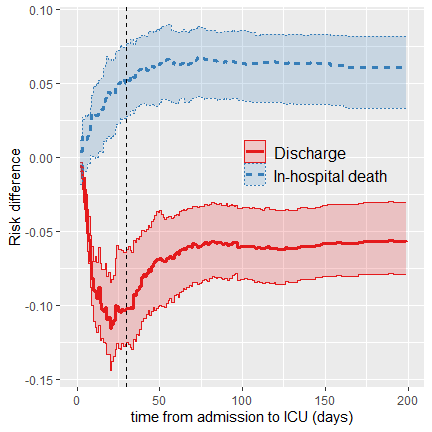Figure 15: Risk differences for discharge and in-hospital death. df <- rbind(summary(res.stab.ATE, event=1, estimand="ATE.RMT"), summary(res.stab.ATE, event=2, estimand="ATE.RMT")) df$Event <- as.factor(df$Event) levels(df$Event) <- c("Discharge", "In-hospital death")

ggplot(df, aes(x=time, y=ATE.RMT, color=Event, fill=Event,
shape=Event, linetype=Event)) + geom_line(linewidth=1.1) +
geom_ribbon(aes(ymin=CIL.ATE.RMT, ymax=CIU.ATE.RMT), alpha=0.2) +
scale_color_brewer(palette = "Set1") +
scale_fill_brewer(palette = "Set1") + xlab("time from admission to ICU (days)") +
ylab("average time (days) lost/gained due to treatment") +
theme(legend.position = c(0.7, 0.5), legend.text=element_text(size=12),
legend.background=element_rect(fill="transparent"), legend.title=element_blank())+
geom_vline(xintercept=30, linetype="dashed")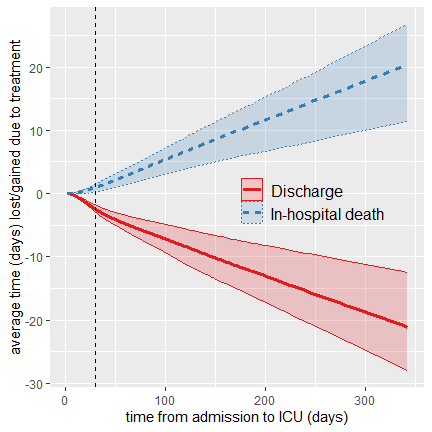Figure 16: Difference in average time lost/gained due to treatment.

### Estimating treatment effect on the composite Length-of-Stay (LOS) for stabilized ATE weights

From the cause-specific parameters we can derive an overall effect of RHC on the composite LOS. Here we focus on the the difference between the cumulative distribution functions of the LOS in two treatment arms:

alpha=0.05
# trt.0:
bs.CumPr.0 <- 1-exp(-res.stab.ATE$trt.0[[paste("Ev=", 1, sep="")]]$bs.CumHaz -
res.stab.ATE$trt.0[[paste("Ev=", 2, sep="")]]$bs.CumHaz)
CumPr.CIL.0 <- apply(bs.CumPr.0, 2, quantile, prob=alpha/2, na.rm=TRUE)
CumPr.CIU.0 <- apply(bs.CumPr.0, 2, quantile, prob=1-alpha/2, na.rm=TRUE)
# trt.1:
bs.CumPr.1 <- 1-exp(-res.stab.ATE$trt.1[[paste("Ev=", 1, sep="")]]$bs.CumHaz -
res.stab.ATE$trt.1[[paste("Ev=", 2, sep="")]]$bs.CumHaz)
CumPr.CIL.1 <- apply(bs.CumPr.1, 2, quantile, prob=alpha/2, na.rm=TRUE)
CumPr.CIU.1 <- apply(bs.CumPr.1, 2, quantile, prob=1-alpha/2, na.rm=TRUE)

df <- rbind(data.frame(time=res.stab.ATE$time, TRT=1, CumPr=1-exp(-res.stab.ATE$trt.1[[paste("Ev=", 1, sep="")]]$CumHaz - res.stab.ATE$trt.1[[paste("Ev=", 2, sep="")]]$CumHaz), CumPr.CIL=CumPr.CIL.1, CumPr.CIU=CumPr.CIU.1), data.frame(time=res.stab.ATE$time, TRT=2,
CumPr=1-exp(-res.stab.ATE$trt.0[[paste("Ev=", 1, sep="")]]$CumHaz -
res.stab.ATE$trt.0[[paste("Ev=", 2, sep="")]]$CumHaz),
CumPr.CIL=CumPr.CIL.0, CumPr.CIU=CumPr.CIU.0))
df$TRT <- as.factor(df$TRT)
levels(df$TRT) <- c("RHC", "No-RHC") ggplot(df, aes(x=time, y=CumPr, color=TRT, fill=TRT, shape=TRT)) + geom_step(linewidth=1.1) + scale_color_brewer(palette = "Set1") + scale_fill_brewer(palette = "Set1") + xlim(0,100) + xlab("time (days)") + ylab("Cumulative distribution of length-of-stay") + geom_ribbon(aes(ymin=CumPr.CIL, ymax=CumPr.CIU), alpha=0.2, stat="stepribbon")+ theme(axis.text.x = element_text(face="bold", angle=45), axis.text.y = element_text(face="bold"), plot.title = element_text(hjust = 0.5))+ theme(legend.position = c(0.6, 0.3), legend.text=element_text(size=12), legend.background=element_rect(fill="transparent"), legend.title=element_blank())+ geom_vline(xintercept=30, linetype="dashed")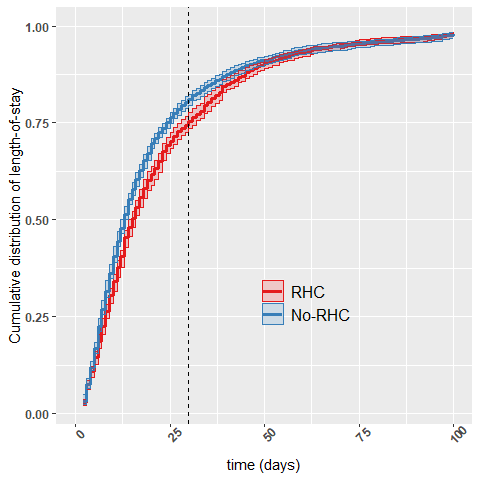Figure 17: Cumulative distribution of length-of-stay in RHC vs No-RHC groups. ## Estimating the effect of RHC on 30-day survival for stabilized ATE weights Here we would really like to know whether the RHC procedure improves short-term 30-day survival, either death happens in the hospital or at home. Our time outcome is the time-to-death, which might be censored at day 30. Below we illustrate how to use causalCmprsk in survival data with only one time-to-event outcome. ### Graphical test of a proportional hazards assumption # nonparametric: res.30 <- fit.nonpar(df=rhc_full, X="T.death.30", E="D.30", A="RHC", C=covs.names, wtype="stab.ATE", cens=0, conf.level=0.95, bs=TRUE, nbs.rep=80, seed=17, parallel = FALSE) # Cox-based estimation: res.cox.30 <- fit.cox(df=rhc_full, X="T.death.30", E="D.30", A="RHC", C=covs.names, wtype="stab.ATE", cens=0, conf.level=0.95, bs=TRUE, nbs.rep=80, seed=17, parallel = FALSE) df1.30 <- cbind(summary(res.30, event=1, estimand="logHR") %>% select(time, logCumHazR, CIL.logCumHazR, CIU.logCumHazR), Est.method=1) HR.30 <- res.cox.30$trt.eff[[paste("Ev=", 1, sep="")]][c("log.CumHazR", "log.CumHazR.CI.L", "log.CumHazR.CI.U")]
df2.30 <- data.frame(time=c(min(res.cox.30$time), max(res.cox.30$time)), logCumHazR=HR.30[],
CIL.logCumHazR=NA, CIU.logCumHazR=NA, Est.method=2)
df.30 <- rbind(df1.30, df2.30)
df.30$Est.method <- factor(df.30$Est.method)
levels(df.30$Est.method) <- c("nonpar", paste("Cox: ", round(res.cox.30$trt.eff[[paste("Ev=", 1, sep="")]]$log.CumHazR,dig=2), ", 95% CI=[", round(res.cox.30$trt.eff[[paste("Ev=", 1, sep="")]]$log.CumHazR.CI.L,dig=2), ",", round(res.cox.30$trt.eff[[paste("Ev=", 1, sep="")]]$log.CumHazR.CI.U,dig=2), "]", sep="")) ggplot(df.30, aes(x=time, y=logCumHazR, color=Est.method, fill=Est.method, shape=Est.method)) + geom_step(linewidth=1.1) + geom_ribbon(aes(ymin=CIL.logCumHazR, ymax=CIU.logCumHazR), alpha=0.2, stat="stepribbon") + scale_color_brewer(palette = "Set1") + scale_fill_brewer(palette = "Set1") + xlab("time from admission to ICU (days)") + ylim(-0.1, 0.55)+ ylab("log-ratio of CumHaz(t)") + theme(axis.text.x = element_text(face="bold", angle=45), axis.text.y = element_text(face="bold"), plot.title = element_text(hjust = 0.5)) + theme(legend.position = c(0.6, 0.7), legend.title=element_blank(), legend.text=element_text(size=12), legend.background=element_rect(fill="transparent"))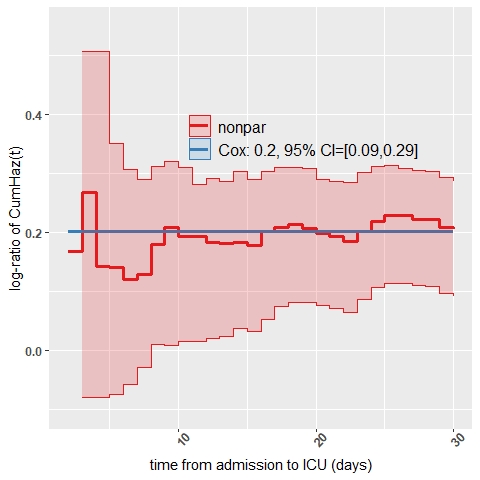Figure 18: log-ratio of cumulative hazards for 30-day mortality, using stabilized ATE weighting. ### Estimating risk difference ggplot(summary(res.30, event=1, estimand="RD"), aes(x=time, y=RD)) + geom_step(linewidth=1.1) + geom_ribbon(aes(ymin=CIL.RD, ymax=CIU.RD), alpha=0.2, stat="stepribbon") + xlab("time from admission to ICU (days)") + ylab("Risk difference") est30 <- get.pointEst(res.30, 30) cat("30-day risk difference=", round((est30$trt.eff[]$RD), dig=4), ", 95% CI=[", round((est30$trt.eff[]$RD.CI.L), dig=4), ", ", round((est30$trt.eff[]$RD.CI.U), dig=4), "]\n", sep="") 30-day risk difference=0.0555, 95% CI=[0.0244, 0.0795]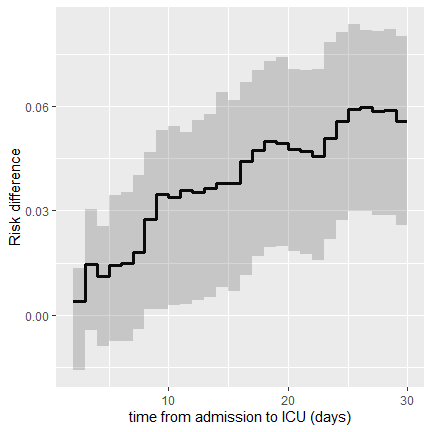Figure 19: Risk difference for 30-day mortality. ### Estimating risk ratio ggplot(summary(res.30, event=1, estimand="RR"), aes(x=time, y=RR)) + geom_step(linewidth=1.1) + geom_ribbon(aes(ymin=CIL.RR, ymax=CIU.RR), alpha=0.2, stat="stepribbon") + xlab("time from admission to ICU (days)") + ylab("Risk ratio") cat("30-day risk ratio=", round((est30$trt.eff[]$RR), dig=4), ", 95% CI=[", round((est30$trt.eff[]$RR.CI.L), dig=4), ", ", round((est30$trt.eff[]$RR.CI.U), dig=4), "]\n", sep="") 30-day risk ratio=1.1783, 95% CI=[1.0763, 1.2518]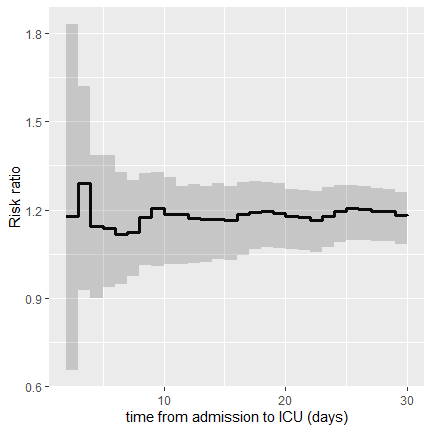Figure 20: Risk ratio for 30-day mortality. ### Estimating difference in RMT The above risk difference can be translated into the domain of the average days lost as a result from undergoing RHC: ggplot(summary(res.30, event=1, estimand="ATE.RMT"), aes(x=time, y=ATE.RMT)) + geom_line(linewidth=1.1) + geom_ribbon(aes(ymin=CIL.ATE.RMT, ymax=CIU.ATE.RMT), alpha=0.2) + xlab("time from admission to ICU (days)") + ylab("RMT difference (days)") cat("30-day RMT difference=", round((est30$trt.eff[]$ATE.RMT), dig=4), ", 95% CI=[", round((est30$trt.eff[]$ATE.RMT.CI.L), dig=4), ", ", round((est30$trt.eff[]\$ATE.RMT.CI.U), dig=4), "]\n", sep="")
30-day RMT difference=1.0847, 95% CI=[0.3561, 1.5656]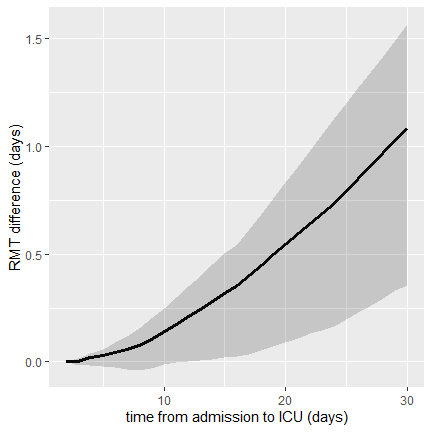Figure 21: Difference in average time lost due to RHC in the 30-day mortality.